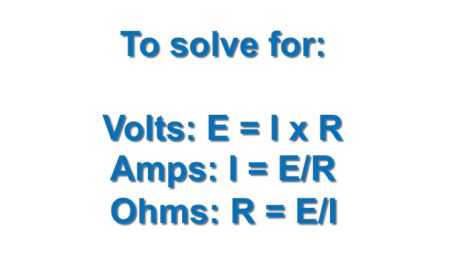Interactive

# Determine Circuit Outputs from Specified Inputs

Martech
Updated Jan 21, 2021

When you complete this lesson, you will be able to use formulas to compute DC series and parallel circuit outputs based on the known inputs. • Compute total current flow through a DC series circuit • Compute total resistance in a DC series circuit • Compute voltage drops across individual resistors in a DC series circuit • Compute total voltage drop through all resistors in a DC series circuit • Compute power dissipated in DC series circuits • Calculate the total power in a DC series circuit • Compute the current in branches of a parallel DC circuit • Compute the total current in a DC parallel circuit • Compute the potential (voltage drop) across resistors in a DC parallel circuit • Compute power dissipated in the resistors of a DC parallel circuit • Compute total power in a DC parallel circuit • Compute the tota

;